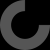MakerBot Print is our newest print-prepration software, which supports native CAD files and STL assemblies,
allows you to interact with all your printers via the Cloud, and many other exciting new features.

by jcernato Jan 11, 2017

Thing Apps Enabled

2019-04-20:

ERROR: Parser error in line 37: syntax error
ERROR: Compilation failed!

at Line:
/ A / for(i=[0:nm]) [(d-k)/2cosinus(n,i),(d-k)/2*sinus(n,i),height(k,n,i,-0.9+off2)],

looking into development version in some days ;)

Love this! Here is a simple example of generating female threads if anyone is looking:

femaleSize = 5.2;
maleSize = 5;
screwHeight=5;
cylinderShell = 2;
cylinderHeight=screwHeight;

// Female
difference() {
cylinder (h = cylinderHeight, r=cylinderRadius, center = false, \$fn=100);
}

// Male

I'm using the nightly(?) build of OpenScad, and I wrote a little program to put a screw in a cylinder. The program works in preview mode, but when rendering, it either errors or just doesn't render the screw... am I doing something wrong?

include

\$fn=60;

module center(){
difference(){
union(){
translate([0,0,-5])
cylinder(h=35,d=3,center=false);
translate([0,0,-5])
}
translate([0,0,-5])
cylinder(h=5,d=28,center=false);
translate([0,0,25])
cylinder(h=5,d=28,center=false);
}
}

difference(){
cylinder(h=25,d=25,center=false);
center();
}

Well it's not entirely your fault... If you look closely into the library you may notice that the coordinates for the "F" and "G" points, which define the inner wall, are set to "d-4*k/2 * ..some_function.." which basically means that it subtracts 4 times the thread_pitch from the diameter. In your case (12-4*4).. results in a negative value.
I've tried to set the x,y coordinates of these points to '0' so that the nasty hole in the middle would be closed all together, sadly this too results in errors...
You can however set the width of the hole to a fixed size, change the lines for these two points by deleting the proportional factor "d-4k/2". Those lines should then read:
/* F */ for(i=[0:n]) [cosinus(n,i),sinus(n,i),height(k,n,i,-0.5)],
/* G */ for(i=[n*(m-1):n*m]) [cosinus(n,i),sinus(n,i),height(k,n,i,+0.5)],
The hole in the center should now always have the same diameter of 2mm (to avoid issues the cylinder in the "union" should be bigger than 2)

Another thing, in your module you cut away the top and bottom of the thread. When you later subtract the module from the bigger cylinder you run into trouble. The object you subtract from the other one should always overlap at least tiny bit.
Your example then can be written much easier:

\$fn=60;
module center(){
union(){
translate([0,0,-5])
cylinder(h=35,d=2.5,center=false);
translate([0,0,-5])
}
}
difference(){
cylinder(h=25,d=25,center=false);
center();
}

or without the 'module' even just:
\$fn=60;
difference(){
cylinder(h=25,d=25,center=false);
translate([0,0,-5]) cylinder(h=35,d=2.5,center=false);
}

thank you! I did get it to work with changing those lines. the module was so I could print the screw, and then print the cylinder with the screw removed, right now it's just a proof of concept though :)

Did a test print for a standard M10 nut used the following.... s_thread(9.5,1.5,15);
And nut fitted first time. (see pic in made)
Thanks again

This is glorious! I have been trying to find an easy way to create 2" BSP . I have a few questions;
How do I create a UNF thread?
I'd like to create a 2" thread with a hole in the center (well another thread so that I can screw in a UNF shaft)? any tips?

thanks for the model!

The trapezoid in the library doesn't follow any standard, you can tweak the shape by adjusting the offset-factors in lines 34-40 (last numbers (-0.4, 0, 0.1, 0.5, -0.5, -0.5, 0.5))
Measurements are in [mm], you'll have to look up the specs for 2"UNF.
Use "difference" to substract the thread from your model, the diameter of the substracted thread has to be bigger though.

difference() {
cylinder(d=80, h=15, \$fn=6);
translate([0,0,-5]) cylinder(d=54, h=25);
}